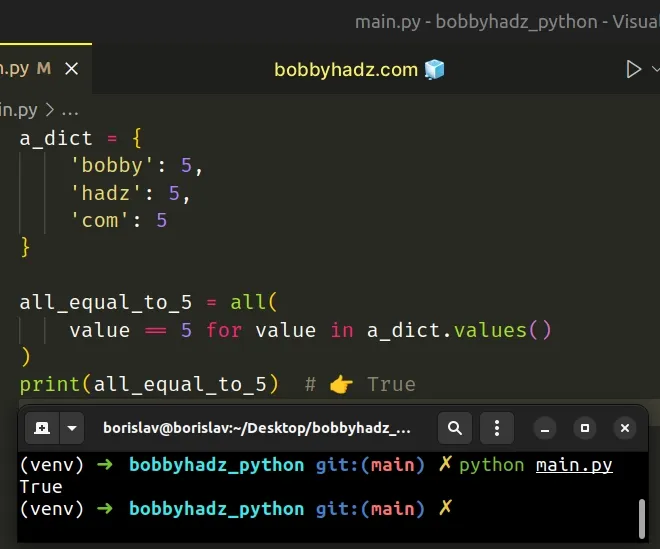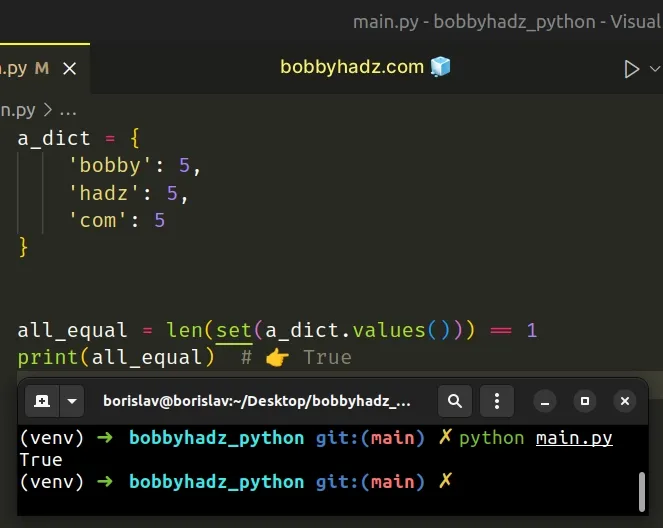# Check if all values in a Dictionary are equal in PythonLast updated: Feb 23, 2023
5 min## #Check if all values in a Dictionary are equal in Python

To check if all values in a dictionary are equal:

1. Use the `dict.values()` method to get the first value of the dictionary.
2. Use the `all()` function to iterate over the dictionary's values.
3. If all other dictionary values are equal to the first value, then the dictionary's values are equal.
main.py
```Copied!```a_dict = {
'bobby': 5,
'com': 5
}

# ✅ Check if all values in dict are equal to a specific value

all_equal_to_5 = all(value == 5 for value in a_dict.values())
print(all_equal_to_5)  # 👉️ True

# -------------------------------------------------

# ✅ Check if all values in dict are equal

first_value = list(a_dict.values())
print(first_value)  # 👉️ 5

all_equal = all(value == first_value for value in a_dict.values())
print(all_equal)  # 👉️ True
``````The first example checks if all of the values in a dictionary are equal to a specific value.

The second example checks if all of the values in a dictionary are equal.

We used the `dict.values()` method to get a view of the dictionary's values.

main.py
```Copied!```a_dict = {
'bobby': 5,
'com': 5
}

# 👇️ dict_values([5, 5, 5])
print(a_dict.values())
``````

The all() built-in function takes an iterable as an argument and returns `True` if all elements in the iterable are truthy (or the iterable is empty).

We passed a generator expression to the `all()` function.

Generator expressions are used to perform some operation for every element or select a subset of elements that meet a condition.

On each iteration, we compare the current dictionary value to the number `5` and return the result.

main.py
```Copied!```a_dict = {
'bobby': 5,
'com': 5
}

all_equal_to_5 = all(value == 5 for value in a_dict.values())
print(all_equal_to_5)  # 👉️ True

if all_equal_to_5:
# 👇️ this runs
print('All values in the dictionary are equal to the specified value')
else:
print('Not all values in the dictionary are equal to the specified value')
``````
The `all()` function returns `True` only if the condition is met for all values in the dictionary.

If the condition isn't met at least once, the `all()` function short-circuits and returns `False`.

You can use the same approach if you simply need to check if all of the values in a dictionary are equal.

main.py
```Copied!```a_dict = {
'bobby': 5,
'com': 5
}

first_value = list(a_dict.values())
print(first_value)  # 👉️ 5

all_equal = all(value == first_value for value in a_dict.values())
print(all_equal)  # 👉️ True
``````

We used the `list()` class to convert the view of the dictionary's values to a list to be able to access the first value.

The last step is to compare the first value to all other values in the dictionary.

Alternatively, you can use a `set` object.

## #Check if all values in a Dictionary are equal using a `set`

This is a three-step process:

1. Use the `set()` class to convert the dictionary's values to a `set` object.
2. Use the `len()` function to get the length of the `set`.
3. If the `set` has a length of `1`, then all values in the dictionary are equal.
main.py
```Copied!```a_dict = {
'bobby': 5,
'com': 5
}

all_equal = len(set(a_dict.values())) == 1
print(all_equal)  # 👉️ True
``````The set() class takes an iterable optional argument and returns a new `set` object with elements taken from the iterable.

Set objects are unordered collections of unique elements, so when we convert the dictionary's values to a `set` all duplicates are automatically removed.

The last step is to use the `len()` function to get the length of the `set` object and compare the result to `1`.

If the `set` has a length of 1, then all values in the dictionary are equal.

Alternatively, you can use the `list.count()` method.

## #Check if all values in a Dictionary are equal using `list.count()`

This is a three-step process:

1. Use the `dict.values()` method to get the first value in the dictionary.
2. Convert the dictionary's values to a list.
3. Use the `list.count()` method to check if the occurrences of the first value are equal to the dictionary's length.
main.py
```Copied!```a_dict = {
'bobby': 5,
'com': 5
}

first_value = list(a_dict.values())

all_equal = list(a_dict.values()).count(first_value) == len(a_dict)
print(all_equal)  # 👉️ True
``````
We used the `list()` class to convert the dictionary's values to a list to be able to use the `list.count()` method.

The `list.count()` method takes a value and returns the number of times the provided value appears in the list.

main.py
```Copied!```my_list = [None, None, None]

print(my_list.count(None))  # 👉️ 3

print(my_list.count('hello'))  # 👉️ 0
``````

If the number of occurrences of the first value in the dictionary is equal to the dictionary's length, then all values in the dictionary are equal.

## #Check if all values in a Dictionary are equal using a `for` loop

This is a four-step process:

1. Use the `dict.values()` method to get a view of the dictionary's values.
2. Use a `for` loop to iterate over the view object.
3. Check if each value is not equal to the first value.
4. Break out of the loop if the condition is met.
main.py
```Copied!```a_dict = {
'bobby': 0,
'com': 0
}

all_values_equal = True

first_value = list(a_dict.values())

for value in a_dict.values():
if value != first_value:
all_values_equal = False
break

print(all_values_equal)  # 👉️ True
``````

We initialized the `all_values_equal` variable to `True`.

On each iteration of the for loop, we check if the current value is not equal to the first value.

If the condition is met, we set the `all_values_equal` variable to `False` and break out of the loop.

The break statement breaks out of the innermost enclosing `for` or `while` loop.

If all of the values in the dictionary are equal, the condition in the `if` statement is never met and the `all_values_equal` variable remains set to `True`.

Which approach you pick is a matter of personal preference. I'd use the `all()` function because I find it quite intuitive and direct.